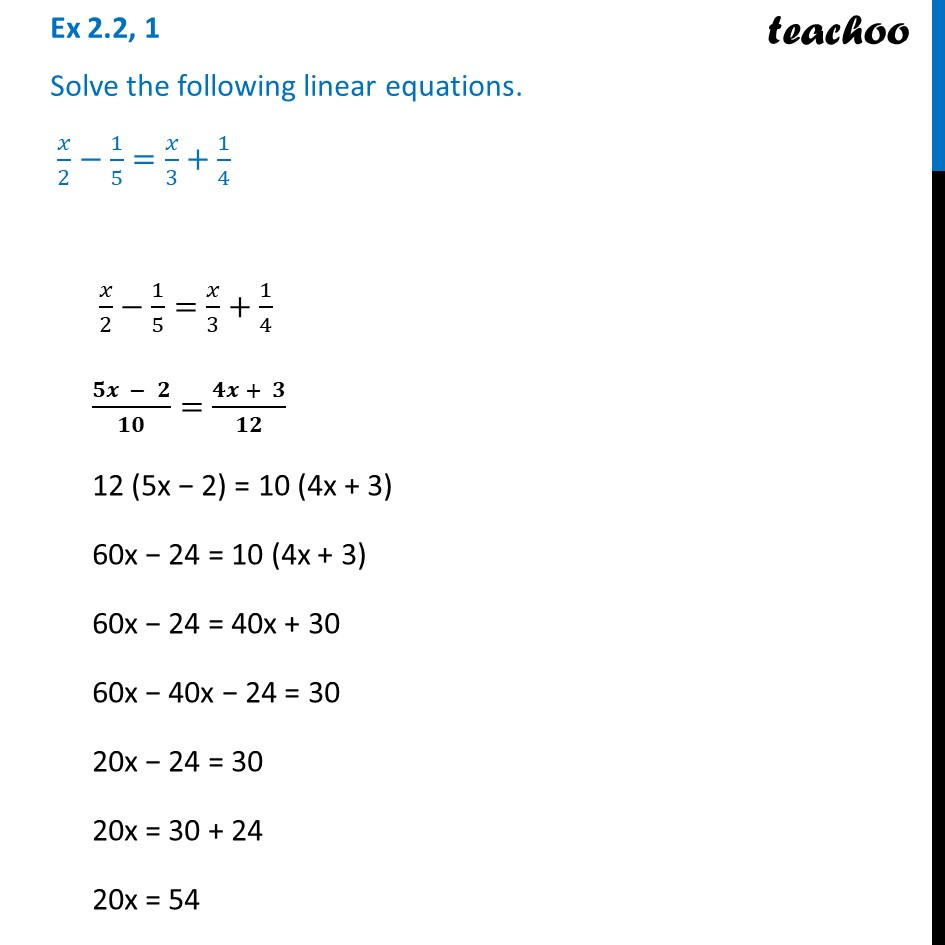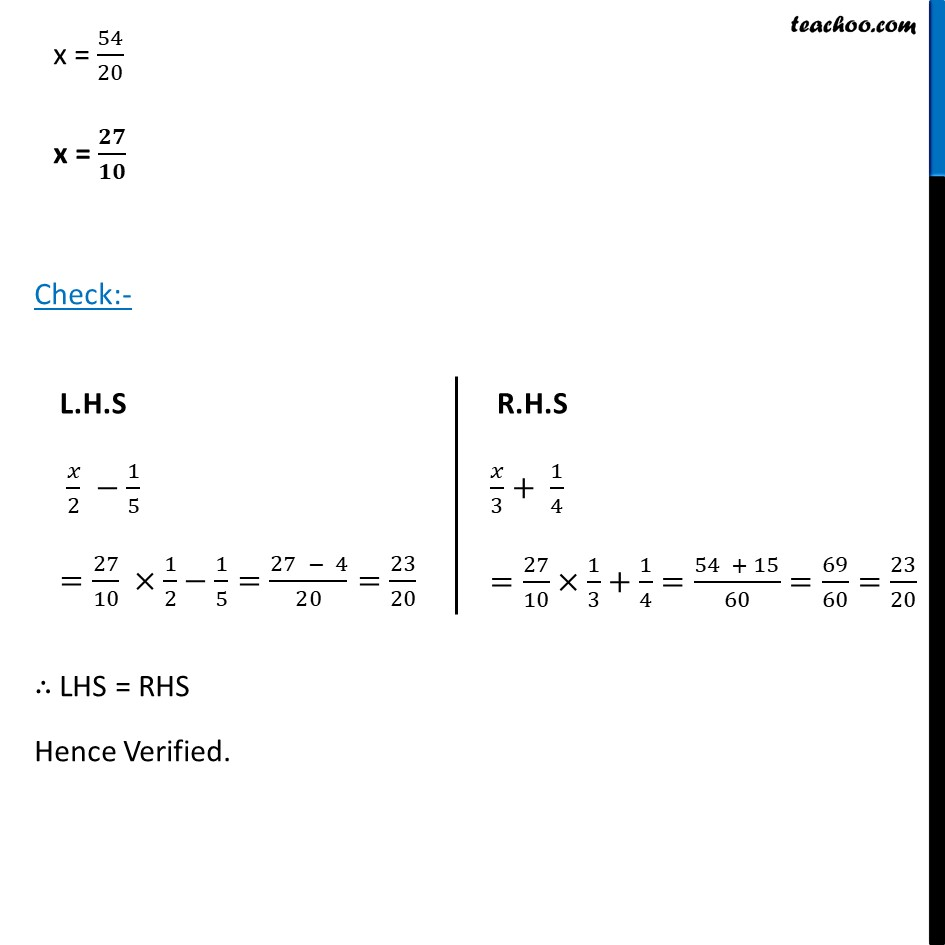Ex 2.2

Chapter 2 Class 8 Linear Equations in One Variable
Serial order wiseLearn in your speed, with individual attention - Teachoo Maths 1-on-1 Class

### Transcript

Ex 2.2, 1 Solve the following linear equations. 𝑥/2−1/5=𝑥/3+1/4 𝑥/2−1/5=𝑥/3+1/4 (𝟓𝒙 − 𝟐)/𝟏𝟎=(𝟒𝒙 + 𝟑)/𝟏𝟐 12 (5x − 2) = 10 (4x + 3) 60x − 24 = 10 (4x + 3) 60x − 24 = 40x + 30 60x − 40x − 24 = 30 20x − 24 = 30 20x = 30 + 24 20x = 54 x = 54/20 x = 𝟐𝟕/𝟏𝟎 Check:- ∴ LHS = RHS Hence Verified. L.H.S 𝑥/2 −1/5 =27/10 ×1/2−1/5=(27 − 4)/20=23/20 R.H.S 𝑥/3+ 1/4 =27/10×1/3+1/4=(54 + 15)/60=69/60=23/20